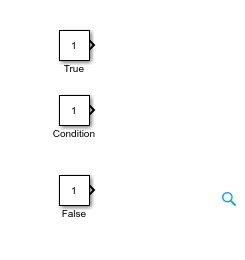# How to use Conditional statements in simulink : tutorial 6

In this tutorial, I will explain you how to work with conditional logical statements in MATLAB Simulink. First of all, a brief and concise introduction of logical statements i.e. IF Else statements is provided, and how we can use them in MATLAB’s simulink. After that the concept provided in introduction part is implemented with the help of MATLAB’s Simulink, and the theoretical results are compared with the virtual results of the block diagram provided by Simulink which comes out to be same. At the end of the tutorial you are provided with an exercise to do it by yourself, and in the next tutorials I will assume that you have done those exercises and I will not explain the concept regarding them.

### Introduction to conditional (IF ELSE) statements

If else statements are used to allows different executions depending on the value of the conditional logic. Based on if the condition given and the input variable matches, the if statements under the if block will be executed and if the condition does not match the else block will be executed.

If you have an experience to work with other programming languages, you must have encountered with conditional statements i.e. If-ELSE statements or IF-THEN-ELSE statement. These statements are used to implement a certain portion of code if a specific condition is true and implement the other portion if that certain condition comes out to be false. Conditional statements are also sometime referred to as conditional expressions or conditional constraints, and are specifically used in cases where we want to execute only selective lines of code.

In Simulink, however, these conditional constraints are referred as switch blocks. Their working depends on a certain condition, which if comes out to be true, will execute one statement, and if turns out to be false, will implement other statement as you will see shortly in the explanation with program portion.

### Example of using if else statements in simulink

Figure 1: Library browser

Figure 2: Constant block search

Figure 3: Constant blocks

• These blocks have different purposes. One of this block is used to store the input which will be displayed at the output if the condition of the conditional block is true. Similarly, one of the block is used to display the output if the condition is false. The third block is a special purpose block and is used as a conditional block. For instance, the condition in the switch block will be verified with respect to this conditional constant block. And condition will be named as true or false depending on this block. Name these blocks according to their working so that they can be recognized in the future as shown in the figure below,Figure 4: Named blocks

Figure 5: Signal routing

Figure 6: Switch block

Figure 7: Connected blocks

Figure 8: Sinks

Figure 9: Display block

Figure 10: Display connected

Figure 11: Threshold condition

Figure 12: Threshold value

Figure 13: True block value

Figure 14: False block value

Figure 15: Block diagram

Figure 16: False output

Figure 17: Conditional constant value

Figure 18: True output

Exercise:

Design a conditional block diagram than will display a 10 at the output if the conditional block is 0 and 100 otherwise.